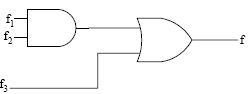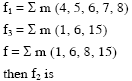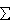# GATE | GATE CS 2008 | Question 85

Given f1, f3 and f in canonical sum of products form (in decimal) for the circuit```A)m(4, 6)

B)m(4, 8)

C)m(6, 8)

D)m(4, 6, 8)```

(A) A
(B) B
(C) C
(D) D

Explanation: From logic diagram  we have f=f1.f2+f3

f=m(4,5,6,7,8).f2+m(1,6,15)—-(1)

from eq(1) we need to find such f2 so that we can get  f=m(1,6,8,15)

eq(1)  says we can get m(1,6,15) from f3 ,so only  8 left

now from option (a,b,d) we get (4,6), (4,8) ,(4,6,8) respectively for m(4,5,6,7,8)f2 which is not required as m4 is undesired.

But option (D) m(4,5,6,7,8)(6,8)+(1,6,15)

• m(6,8)+m(1,6,15)
• m(1,6,8,15)an

s is  ( C) part.

Quiz of this Question

My Personal Notes arrow_drop_up

Article Tags :

Be the First to upvote.

Please write to us at contribute@geeksforgeeks.org to report any issue with the above content.